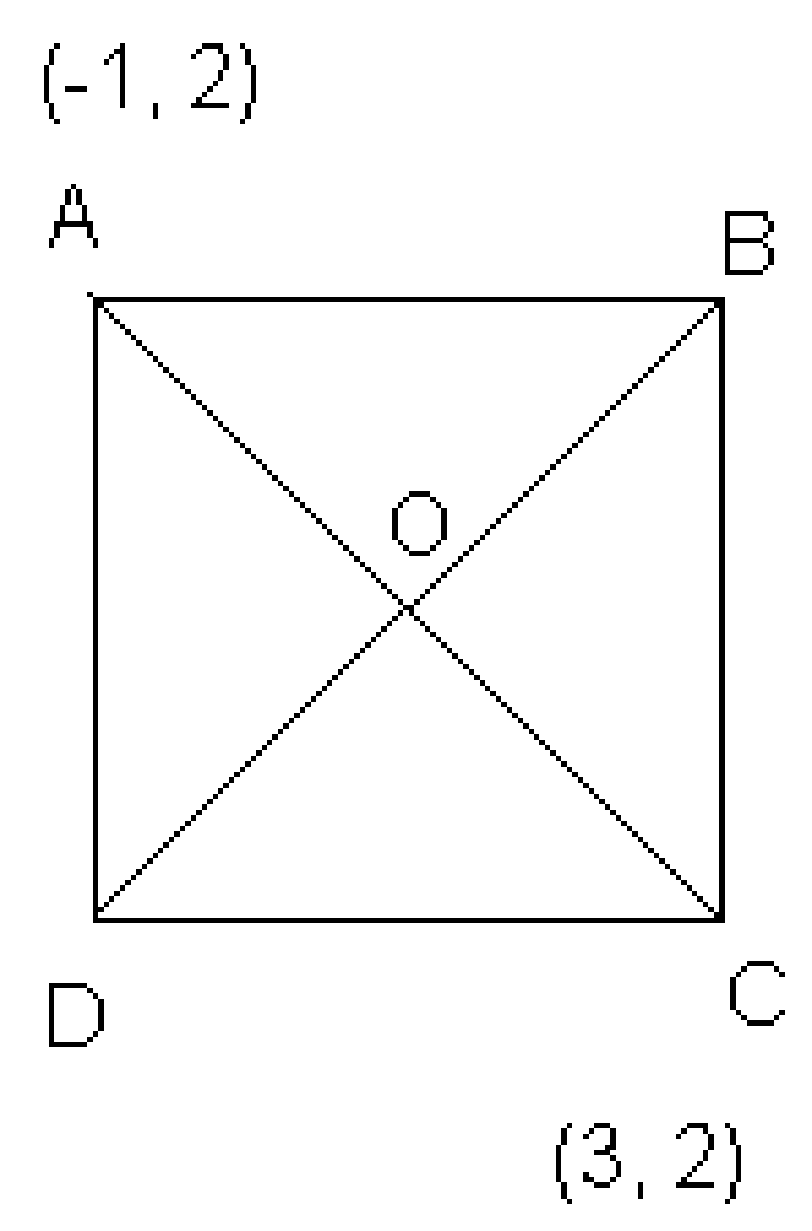Newbie

# The two opposite vertices of a square are (-1, 2) and (3, 2). Find the coordinates of the other two vertices. Q.4

• 0

What is the best solution for the coordinate geometry questions , find the best and simple way to solve the coordinate geometry questions . The two opposite vertices of a square are (-1, 2) and (3, 2). Find the coordinates of the other two vertices.

Share

1. Let ABCD is a square, where A(-1,2) and B(3,2). And Point O is the point of intersection of AC and BD

To Find: Coordinate of points B and D.Step 1: Find distance between A and C and coordinates of point O.

We know that, diagonals of a square are equal and bisect each other.

AC = √[(3 + 1)+ (2 – 2)2] = 4

Coordinates of O can be calculated as follows:

x = (3 – 1)/2 = 1 and y = (2 + 2)/2 = 2

So, O(1,2)

Step 2: Find the side of the square using Pythagoras theorem

Let a be the side of square and AC = 4

From right triangle, ACD,

a = 2√2

Hence, each side of square = 2√2

Step 3: Find coordinates of point D

Equate length measure of AD and CD

Say, if coordinate of D are (x1, y1)

AD = √[(x1 + 1)+ (y1 – 2)2]

Squaring both sides,

AD2 = (x1 + 1)+ (y1 – 2)2

Similarly, CD2 = (x1 – 3)+ (y1 – 2)2

Since all sides of a square are equal, which means AD = CD

(x1 + 1)+ (y1 – 2)2 = (x1 – 3)+ (y1 – 2)2

x12 + 1 + 2x1 = x12 + 9 – 6x1

8x1 = 8

x1 = 1

Value of y1 can be calculated as follows by using the value of x.

From step 2: each side of square = 2√2

CD2 = (x1 – 3)+ (y1 – 2)2

8 = (1 – 3)+ (y1 – 2)2

8 = 4 + (y1 – 2)2

y1 – 2 = 2

y1 = 4

Hence, D = (1, 4)

Step 4: Find coordinates of point B

From line segment, BOD

Coordinates of B can be calculated using coordinates of O; as follows:

Earlier, we had calculated O = (1, 2)

Say B = (x2, y2)

For BD;

1 = (x2 + 1)/2

x= 1

And 2 = (y2 + 4)/2

=> y2 = 0

Therefore, the coordinates of required points are B = (1,0) and D = (1,4)

• -1